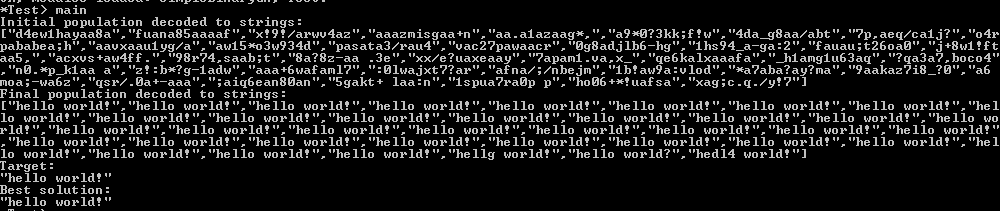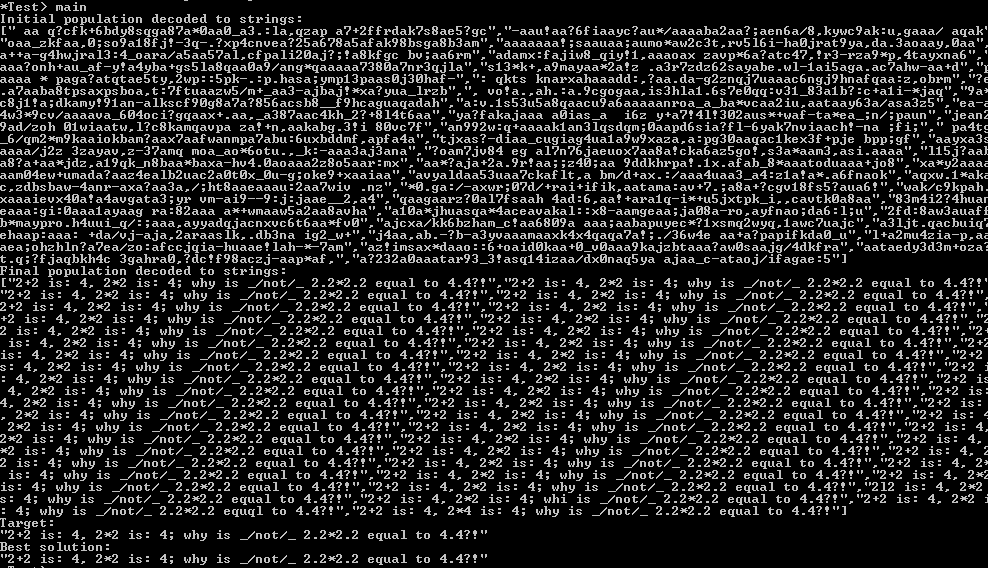Haskell is a purely functional programming language.I have used Haskell to write a simple Binary Genetic Algorithm.Genetic algorithm is optimisation and search technique based on principles of genetics and natural selection.The steps I followed for Binary Genetic Algorithm are :

1. Define cost function, cost, variables.
2. Select GA parameters.
3. Generate initial population.
4. Decode chromosomes.
5. Find cost for each chromosome.
6. Select mates for reproduction.
7. Mating.
8. Mutation.
9. Check stopping criteria • IF (reached max number of iterations OR converged) THEN stop • ELSE go to Step 4.

I have upload the Haskell Script with this post below. [caption id=”attachment_12200” align=”aligncenter” width=”504”]Binary Genetic Algorithm Hello World Haskell[/caption] The algorithm works pretty well for “Hello World”. Only last three guess is wrong. Now lets give complex targetThe algorithm works well for complex combination of characters also. You can try the different target combination changing in SimpleBinaryGA.hs Click Here to download Source Code Credit:Robin Bye